Skip to content
Related Articles
Classifying data using Support Vector Machines(SVMs) in R
• Last Updated : 28 Aug, 2018

In machine learning, Support vector machine(SVM) are supervised learning models with associated learning algorithms that analyze data used for classification and regression analysis. It is mostly used in classification problems. In this algorithm, each data item is plotted as a point in n-dimensional space (where n is number of features), with the value of each feature being the value of a particular coordinate. Then, classification is performed by finding the hyper-plane that best differentiates the two classes.

In addition to performing linear classification, SVMs can efficiently perform a non-linear classification, implicitly mapping their inputs into high-dimensional feature spaces.

### How SVM works

A Support Vector Machine (SVM) is a discriminative classifier formally defined by a separating hyperplane. In other words, given labeled training data (supervised learning), the algorithm outputs an optimal hyperplane which categorizes new examples.

The most important question that arise while using SVM is how to decide right hyper plane. Consider the following scenarios:

• Scenario 1:
In this scenario there are three hyper planes called A,B,C. Now the problem is to identify the right hyper-plane which best differentiates the stars and the circles.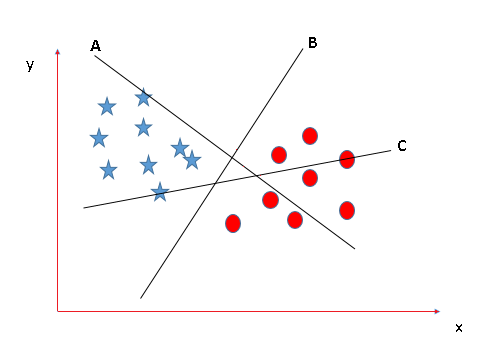The thumb rule to be known, before finding the right hyper plane, to classify star and circle is that the hyper plane should be selected which segregate two classes better.

In this case B classify star and circle better, hence it is right hyper plane.

• Scenario 2:
Now take another Scenario where all three planes are segregating classes well. Now the question arises how to identify the right plane in this situation.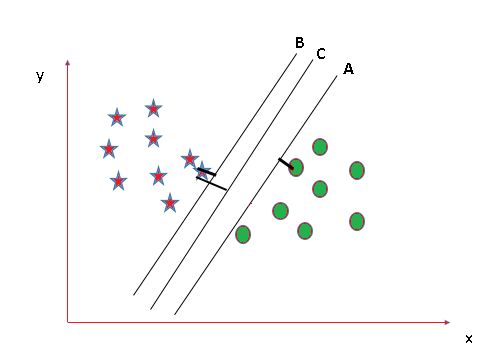In such scenarios, calculate the margin which is the distance between nearest data point and hyper-plane. The plane having the maximum distance will be considered as the right hyper plane to classify the classes better.

Here C is having the maximum margin and hence it will be considered as right hyper plane.

Above are some scenario to identify the right hyper-plane.

Note: For details on Classifying using SVM in Python, refer Classifying data using Support Vector Machines(SVMs) in Python

### Implementation of SVM in R

Here, an example is taken by importing a dataset of Social network aids from file Social.csv

The implementation is explained in the following steps:

• Importing the dataset
 `# Importing the dataset``dataset = ``read.csv``(``'Social_Network_Ads.csv'``)``dataset = dataset[3:5]`

Output: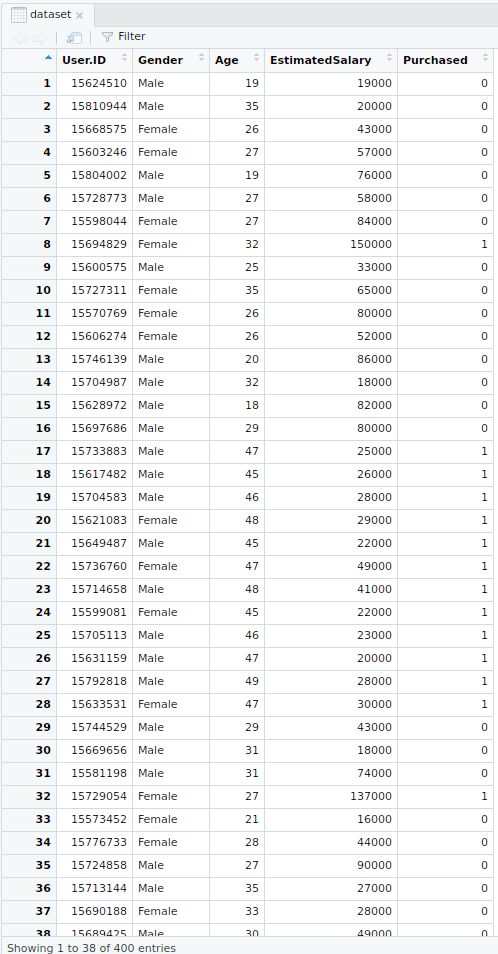• Selecting columns 3-5
This is done for the ease of computation and implementation (to keep the example simple).
 `# Taking columns 3-5``dataset = dataset[3:5]`

Output: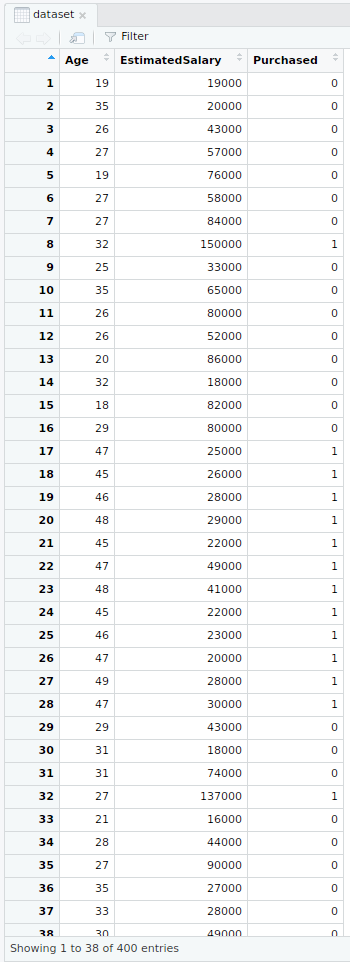• Encoding the target feature
 `# Encoding the target feature as factor``dataset\$Purchased = ``factor``(dataset\$Purchased, levels = ``c``(0, 1))`

Output: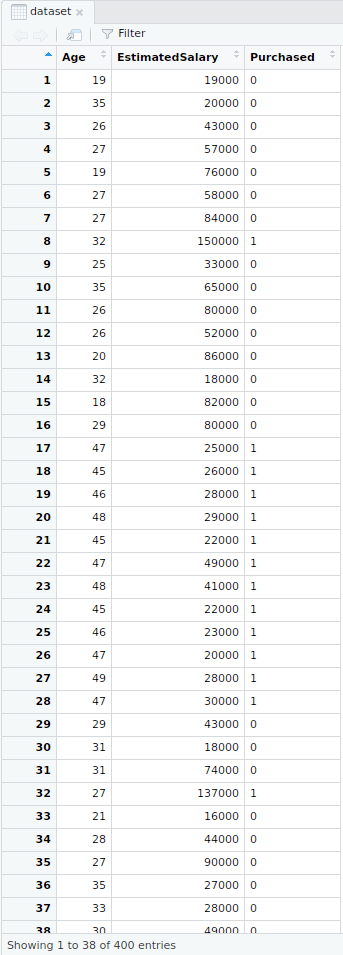• Splitting the dataset
 `# Splitting the dataset into the Training set and Test set``install.packages``(``'caTools'``)``library``(caTools)`` ` `set.seed``(123)``split = ``sample.split``(dataset\$Purchased, SplitRatio = 0.75)`` ` `training_set = ``subset``(dataset, split == ``TRUE``)``test_set = ``subset``(dataset, split == ``FALSE``)`

Output:

• Splitter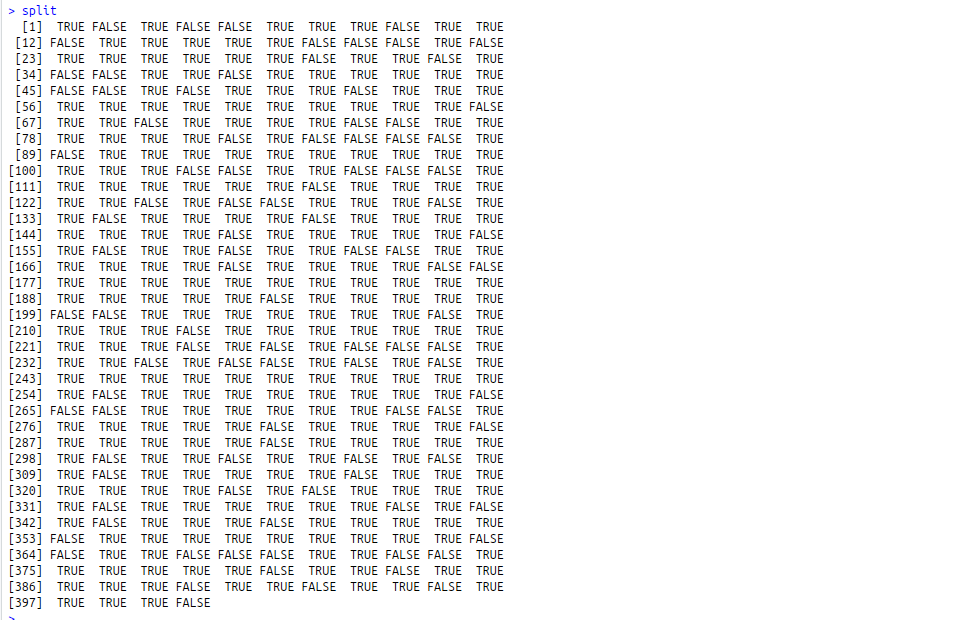• Training dataset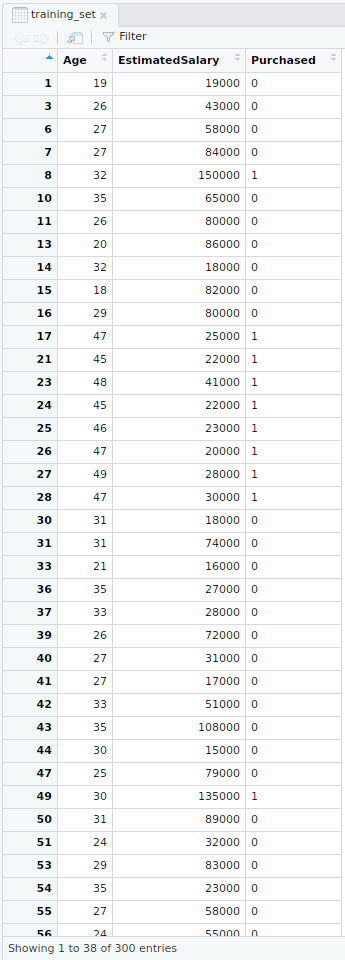• Test dataset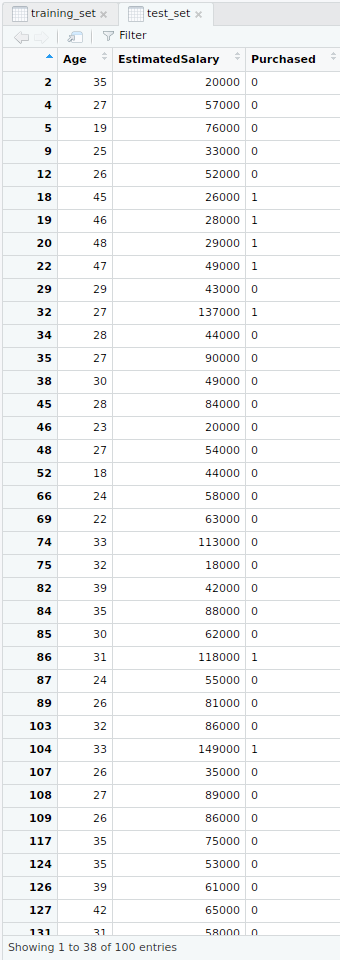• Feature Scaling
 `# Feature Scaling``training_set[-3] = ``scale``(training_set[-3])``test_set[-3] = ``scale``(test_set[-3])`

Output:

• Feature scaled training dataset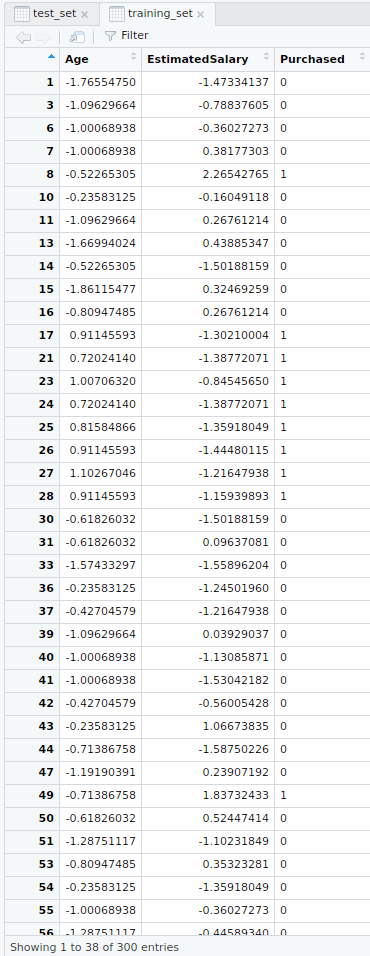• Feature scaled test dataset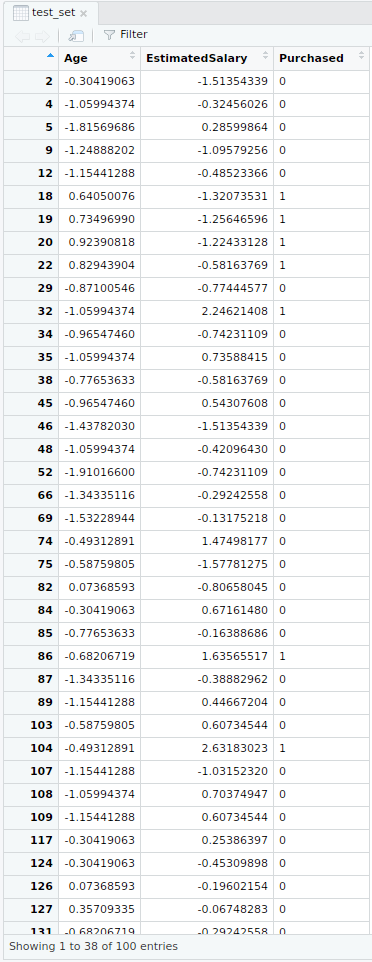• Fitting SVM to the training set
 `# Fitting SVM to the Training set``install.packages``(``'e1071'``)``library``(e1071)`` ` `classifier = ``svm``(formula = Purchased ~ .,``                 ``data = training_set,``                 ``type = ``'C-classification'``,``                 ``kernel = ``'linear'``)`

Output:

• Classifier detailed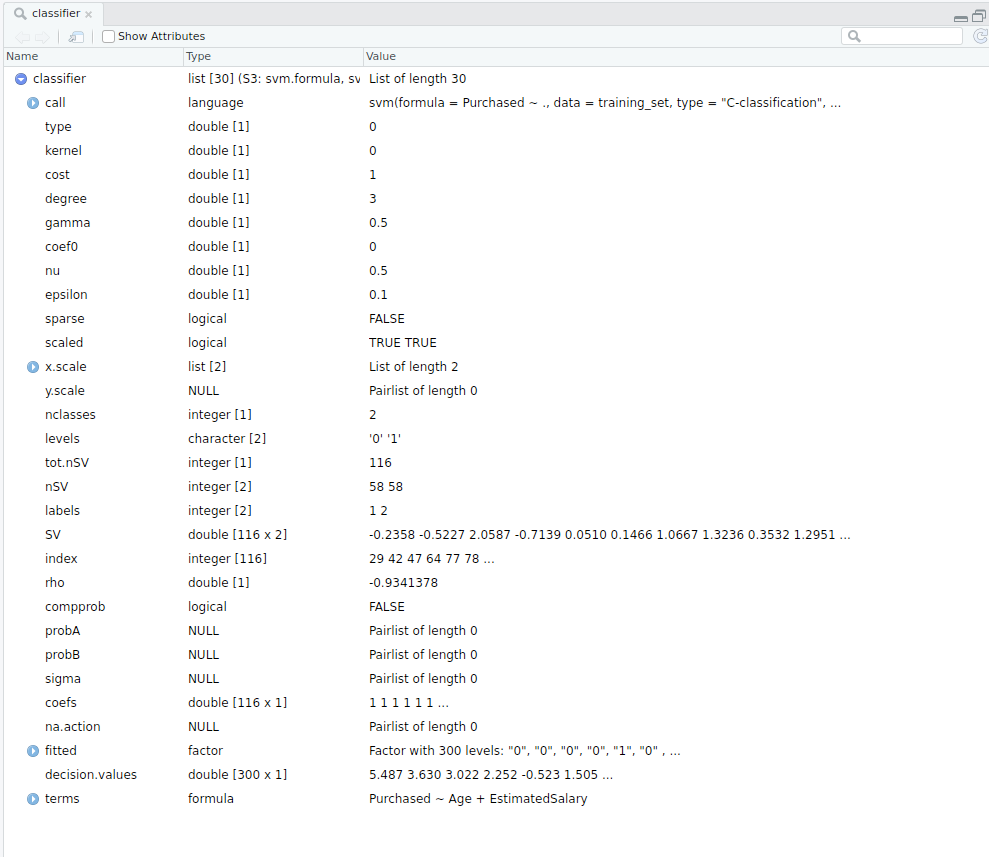• Classifier in nutshell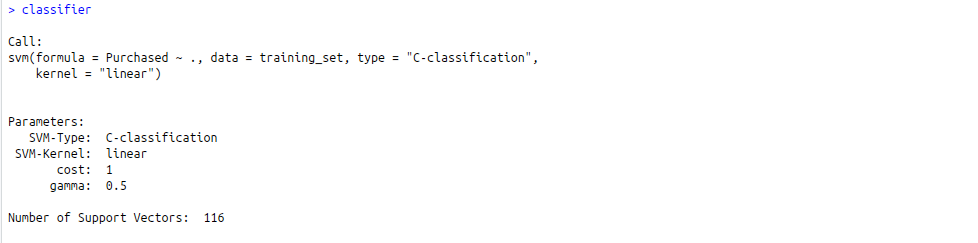• Predicting the test set result
 `# Predicting the Test set results``y_pred = ``predict``(classifier, newdata = test_set[-3])`

Output: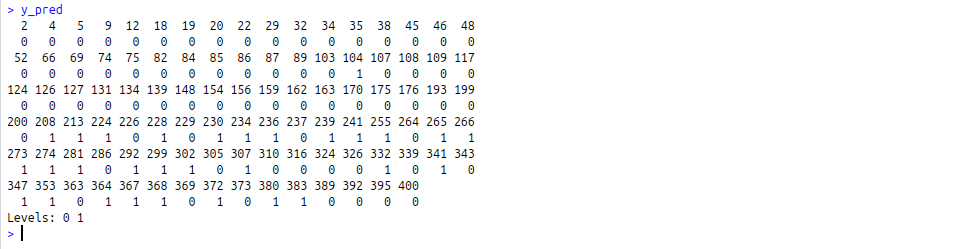• Making Confusion Matrix
 `# Making the Confusion Matrix``cm = ``table``(test_set[, 3], y_pred)`

Output: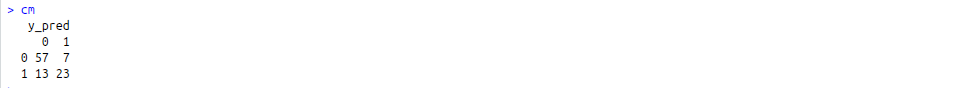• Visualizing the Training set results
 `# installing library ElemStatLearn``library``(ElemStatLearn)`` ` `# Plotting the training data set results``set = training_set``X1 = ``seq``(``min``(set[, 1]) - 1, ``max``(set[, 1]) + 1, by = 0.01)``X2 = ``seq``(``min``(set[, 2]) - 1, ``max``(set[, 2]) + 1, by = 0.01)`` ` `grid_set = ``expand.grid``(X1, X2)``colnames``(grid_set) = ``c``(``'Age'``, ``'EstimatedSalary'``)``y_grid = ``predict``(classifier, newdata = grid_set)`` ` `plot``(set[, -3],``     ``main = ``'SVM (Training set)'``,``     ``xlab = ``'Age'``, ylab = ``'Estimated Salary'``,``     ``xlim = ``range``(X1), ylim = ``range``(X2))`` ` `contour``(X1, X2, ``matrix``(``as.numeric``(y_grid), ``length``(X1), ``length``(X2)), add = ``TRUE``)`` ` `points``(grid_set, pch = ``'.'``, col = ``ifelse``(y_grid == 1, ``'coral1'``, ``'aquamarine'``))`` ` `points``(set, pch = 21, bg = ``ifelse``(set[, 3] == 1, ``'green4'``, ``'red3'``))`

Output: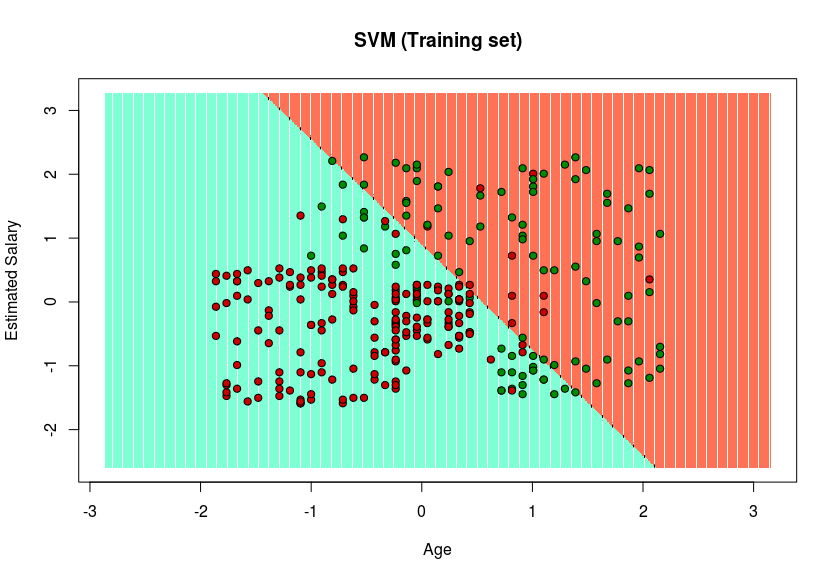• Visualizing the Test set results
 `set = test_set``X1 = ``seq``(``min``(set[, 1]) - 1, ``max``(set[, 1]) + 1, by = 0.01)``X2 = ``seq``(``min``(set[, 2]) - 1, ``max``(set[, 2]) + 1, by = 0.01)`` ` `grid_set = ``expand.grid``(X1, X2)``colnames``(grid_set) = ``c``(``'Age'``, ``'EstimatedSalary'``)``y_grid = ``predict``(classifier, newdata = grid_set)`` ` `plot``(set[, -3], main = ``'SVM (Test set)'``,``     ``xlab = ``'Age'``, ylab = ``'Estimated Salary'``,``     ``xlim = ``range``(X1), ylim = ``range``(X2))`` ` `contour``(X1, X2, ``matrix``(``as.numeric``(y_grid), ``length``(X1), ``length``(X2)), add = ``TRUE``)`` ` `points``(grid_set, pch = ``'.'``, col = ``ifelse``(y_grid == 1, ``'coral1'``, ``'aquamarine'``))`` ` `points``(set, pch = 21, bg = ``ifelse``(set[, 3] == 1, ``'green4'``, ``'red3'``))`

Output: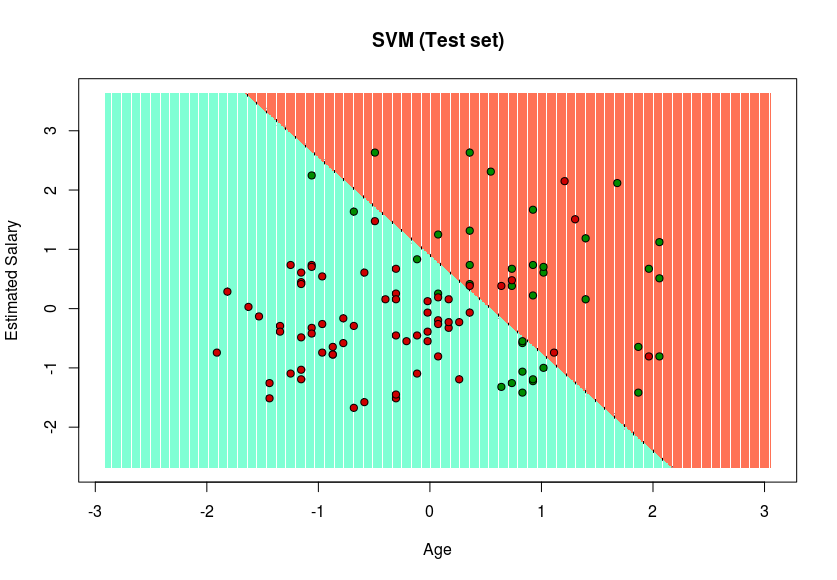Since in the result, a hyper-plane has been found in the Training set result and verified to be the best one in the Test set result. Hence, SVM has been successfully implemented in R.My Personal Notes arrow_drop_up